## 信息含量#

• 市场因素， 所有上市公司都处于相同的宏观经济环境、风险因素和政治、政策背景之下；
• 行业因素， 同一行业中的各上市公司又面临着相似的产业政策、竞争环境和市场特征。

### 样本选择和处理#

（ 1 ）剔除金融行业、 ST 和 *ST 类企业， 以及上市时间不足一年的企业。

（ 2 ）从 MD&A 的内容中分别提取回顾和展望部分， 保存为回顾信息文件和展望信息文件， 部分无法抓取出的年报通过手工收集处理。

（ 3 ）文本处理-文本向量化。 借鉴 Hanley and Hoberg （ 2010 ）的研究思路， 将每个 MD&A 文本通过向量的 形式表示出来， 其每个元素为文本中的每个词语出现的频率。 例如， 假设某 MD&A 文本中包含 10000 个词， 则该文本对应一个 10000×1 维的向量。 举一个简单的例子来描述文本向量化的过程： 在两个简化的 MD&A 文本中， 一个包含“我们生产土豆和生产玉米”， 另一个包含“我们生产家具”， 剔除连词“和”、代词“我们”之后， 只剩下“生产”、“土豆”、“玉米”、“家具”这 4 个词。 那么， 在第一个 MD&A 文本中， “生产”、“土豆”和“玉米”分别出现了 2 次、 1 次和 1 次， 而“家具”出现 0 次， 所以该 文本的向量为 {2 ， 1 ， 1 ， 0} ， 同样得到第二个文本的向量为 {1 ， 0 ， 0 ， 1} 。

（ 4 ）向量标准化。 对于向量化的文本， 仍需解决文本长度不同导致的结果不可比问题。 一般来说， 某一个词在长文本中重复出现的次数较多， 在短文本中重复出现的次数较少， 但并不能因此说 长文本比短文本的信息量大。 为此， 本文进一步将这些向量进行标准化处理， 即将该向量除以文本 中单词的总数， 得到标准化后的向量。 在上面的例子中， 两个公司的标准化之后的向量就成为了 {0.50 ， 0.25 ， 0.25 ， 0} 和 {0.50 ， 0 ， 0 ， 0.50} 。


### 文件目录#

管理层讨论信息含量/
├── 信息含量计算代码.ipynb
├── data/
│   ├── 行业代码.xlsx
│   └── mda10-20.xlsx
├── mda_infor.csv
├── output/
│   └── 2020/
│       ├── 000002.csv
│       ├── 000004.csv
│       ├── 000005.csv
│       ├── 000006.csv
│       ├── ...
│   └── 2019/
│       ├── 000002.csv
│       ├── 000004.csv
│       ├── 000005.csv
│       ├── 000006.csv
│       ├── ...
│   └── 2018/
│       ├── 000002.csv
│       ├── 000004.csv
│       ├── 000005.csv
│       ├── 000006.csv
│       ├── 000006.csv
│       ├── ...
│   └── ...


## 一、导入数据#

import pandas as pd

# converters 强制声明该列为字符串，  防止股票代码 被程序识别为数字，
# 完整数据370+M

#上市公司行业信息

df = pd.merge(df, ind_info_df, on=['股票代码', '会计年度'], how='inner')

#显示前5行


Run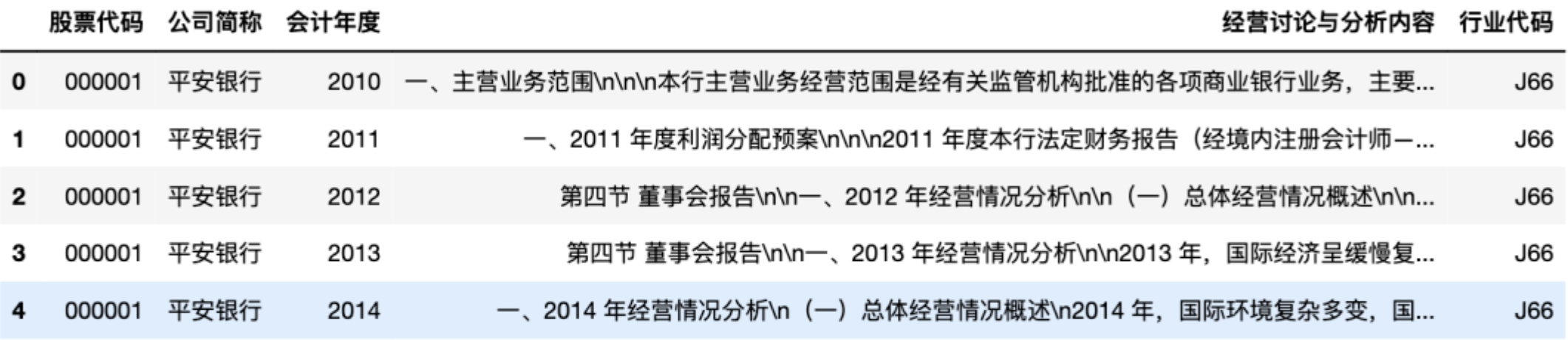# 剔除金融行业处理
df = df[~df['行业代码'].str.contains("J")]
df = df[~df['公司简称'].str.contains("ST")]



Run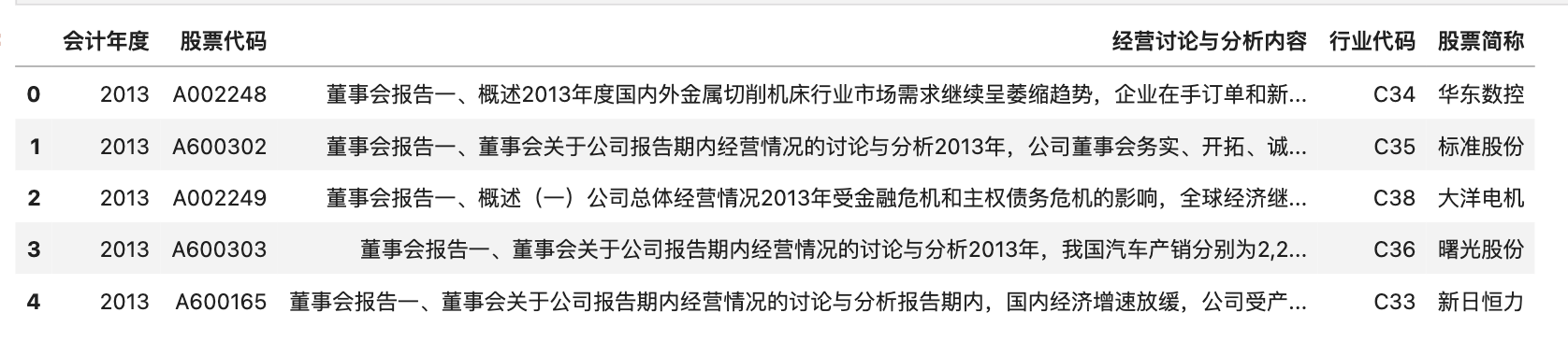## 二、以2020年为例#

1. 选定某年份，以2020年为例
2. 定义transform函数，用于处理「经营讨论与分析内容」字段内的内容。
3. 文本向量化，向量标准化。

### 2.1 选定2020年#

df_per_year = df[df['会计年度']==2020]
df_per_year.reset_index(inplace=True)


Run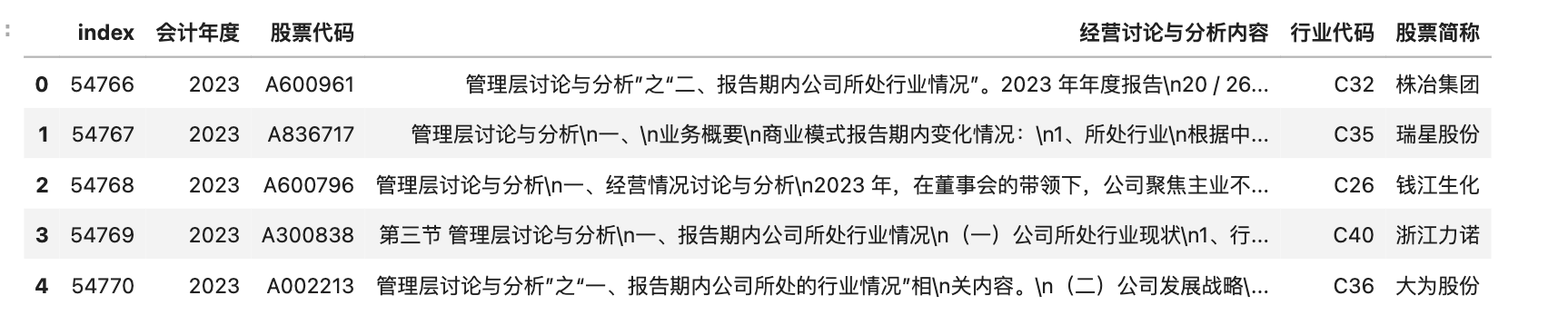### 2.2 定义transform函数#

1. 只保留中文内容
2. 剔除停用词
3. 整理为用空格间隔的字符串(类西方语言文本格式)

import cntext as ct
import jieba
import re

def transform(text):
#只保留md&a中的中文内容
text = ''.join(re.findall('[\u4e00-\u9fa5]+', text))
#剔除停用词
words = [w for w in jieba.cut(text) if w not in stopwords]
#整理为用空格间隔的字符串(类西方语言文本格式)
return ' '.join(words)

df_per_year['clean_text'] = df_per_year['经营讨论与分析内容'].apply(transform)

Building prefix dict from the default dictionary ...
Prefix dict has been built successfully.


### 2.3 文本向量化#

1. 文本向量化
2. 向量标准化
3. 合并多个字段为新的df

from sklearn.feature_extraction.text import CountVectorizer

cv = CountVectorizer(min_df=0.05, max_df=0.5)
# 生成稀疏bow矩阵
dtm_per_year = cv.fit_transform(df_per_year['clean_text'])
dtm_per_year = pd.DataFrame(dtm_per_year.toarray())
dtm_per_year


Run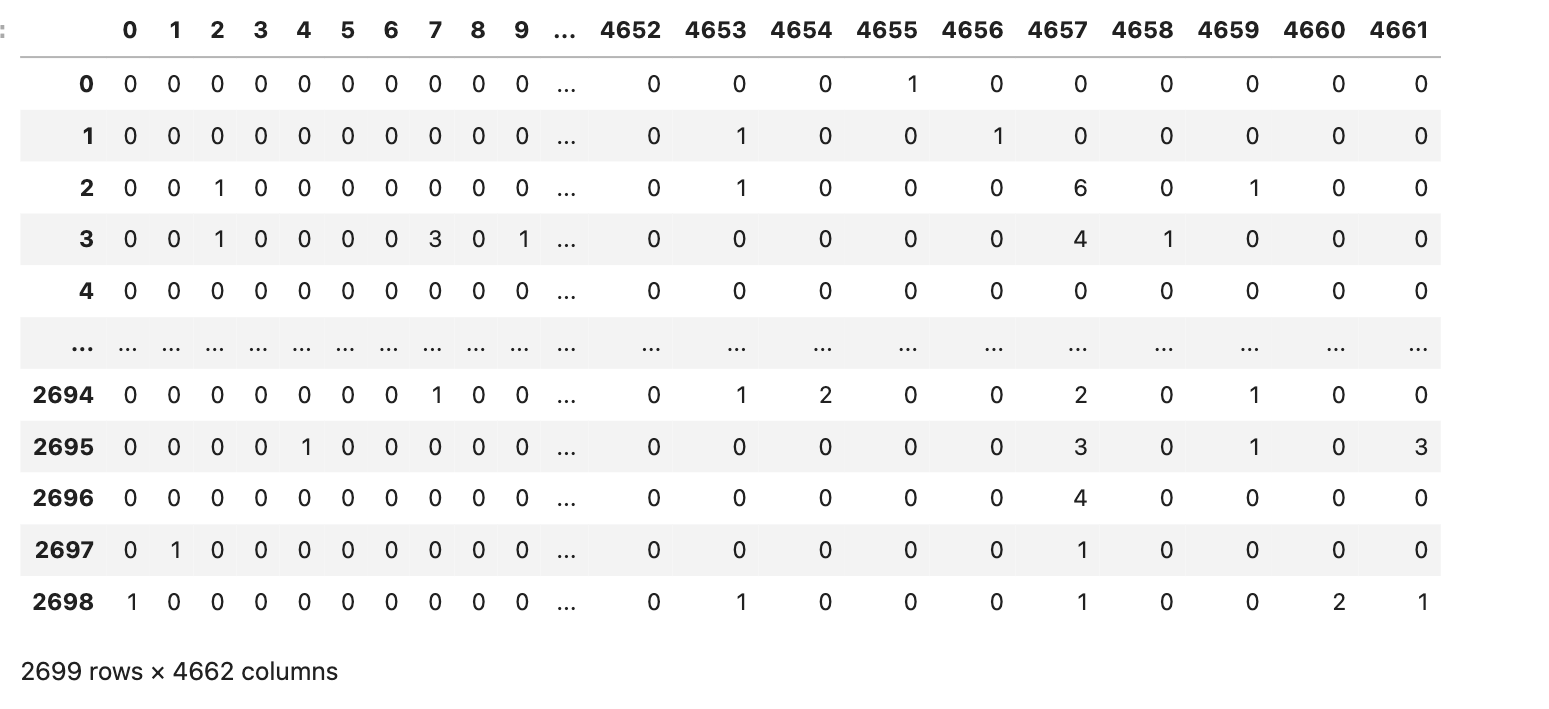import numpy as np

#向量标准化
dtm_per_year = dtm_per_year.apply(lambda row: row/np.sum(row), axis=1)
dtm_per_year


Run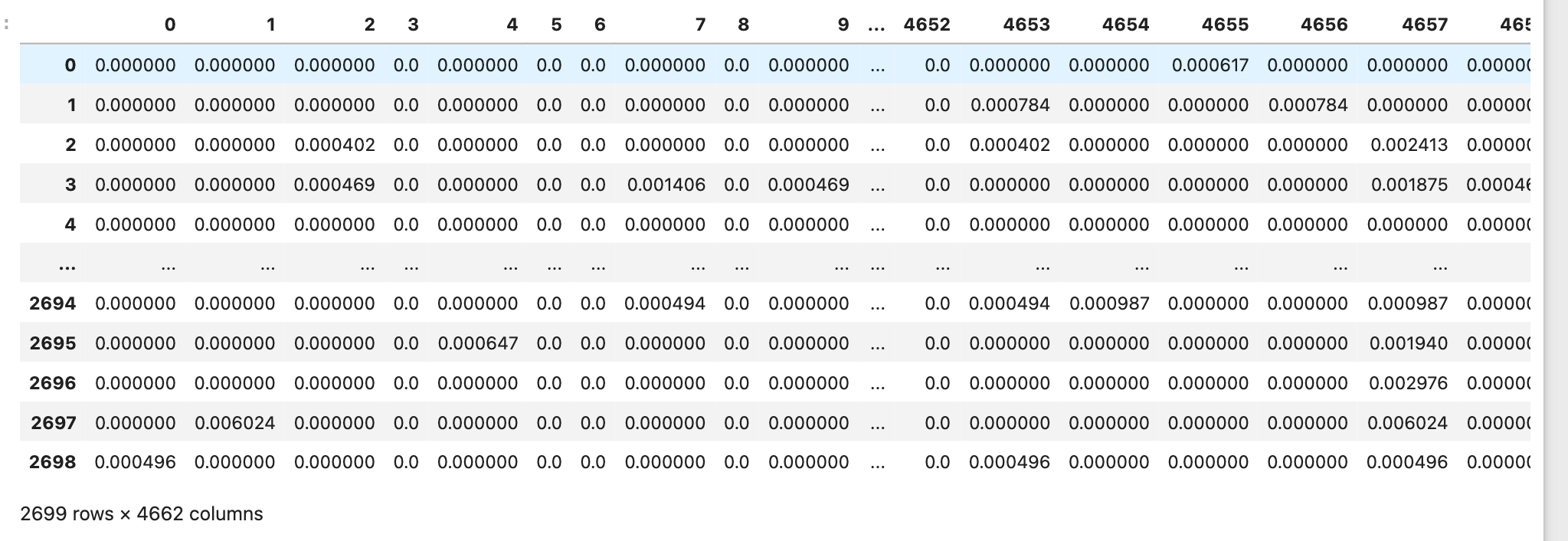#合并多个字段为新的df
dtm_per_year = pd.concat([df_per_year[['股票代码', '会计年度', '行业代码']], dtm_per_year], axis=1)


Run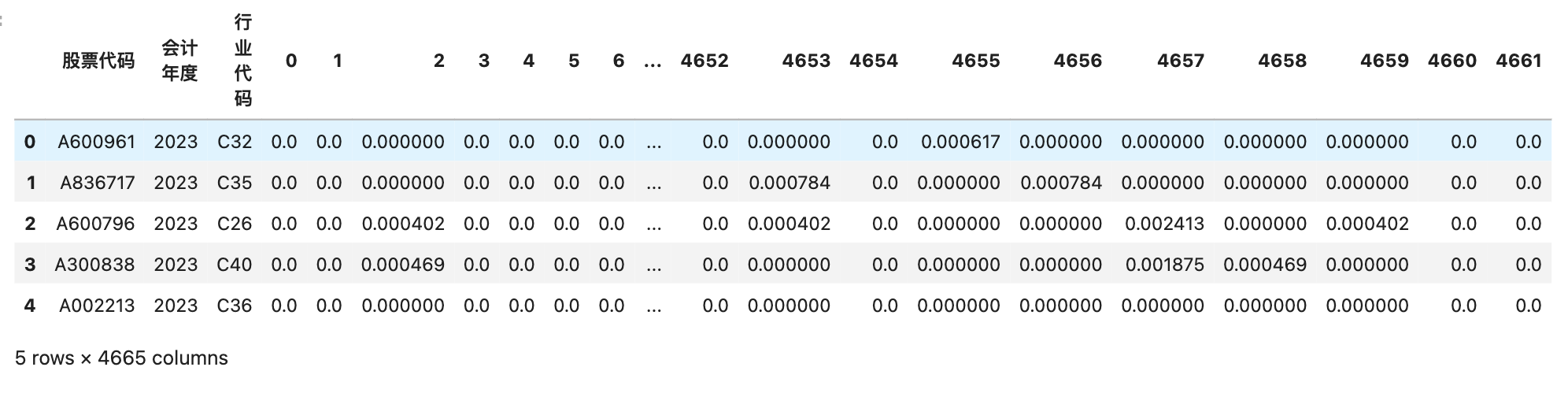## 三、计算2020年行业向量、市场向量#

import os
import pandas as pd

for idx in range(len(dtm_per_year)):
code = dtm_per_year.loc[idx, '股票代码']
ind = dtm_per_year.loc[idx, '行业代码']
year = dtm_per_year.loc[idx, '会计年度']

ind_freq = dtm_per_year[dtm_per_year['行业代码']==ind][dtm_per_year['股票代码']!=code].iloc[:, 3:].mean(axis=0)
market_freq = dtm_per_year[dtm_per_year['行业代码']!=ind].iloc[:, 3:].mean(axis=0)

dtm_per_year_melted = dtm_per_year.melt(id_vars=['股票代码', '会计年度', '行业代码'],
var_name='word_id',
value_name='word_freq')
corporate_df = pd.DataFrame({'word_id': dtm_per_year_melted[dtm_per_year_melted['股票代码']==code]['word_id'].values,
'word_freq': dtm_per_year_melted[dtm_per_year_melted['股票代码']==code]['word_freq'].values,
'ind_freq': ind_freq,
'market_freq':market_freq})
corporate_df['股票代码'] = code
corporate_df['行业代码'] = ind
corporate_df['会计年度'] = year
corporate_df.reset_index(inplace=True)
corporate_df = corporate_df[['股票代码', '行业代码', '会计年度', 'word_id', 'word_freq', 'ind_freq', 'market_freq']]
if not os.path.exists('output/{year}'.format(year=year)):
os.mkdir('output/{year}'.format(year=year))
corporate_df.to_csv('output/{year}/{code}.csv'.format(year=year, code=code), index=False)


## 四、计算2010-2020年所有公司行业向量、市场向量#

• 市场因素， 所有上市公司都处于相同的宏观经济环境、风险因素和政治、政策背景之下；
• 行业因素， 同一行业中的各上市公司又面临着相似的产业政策、竞争环境和市场特征。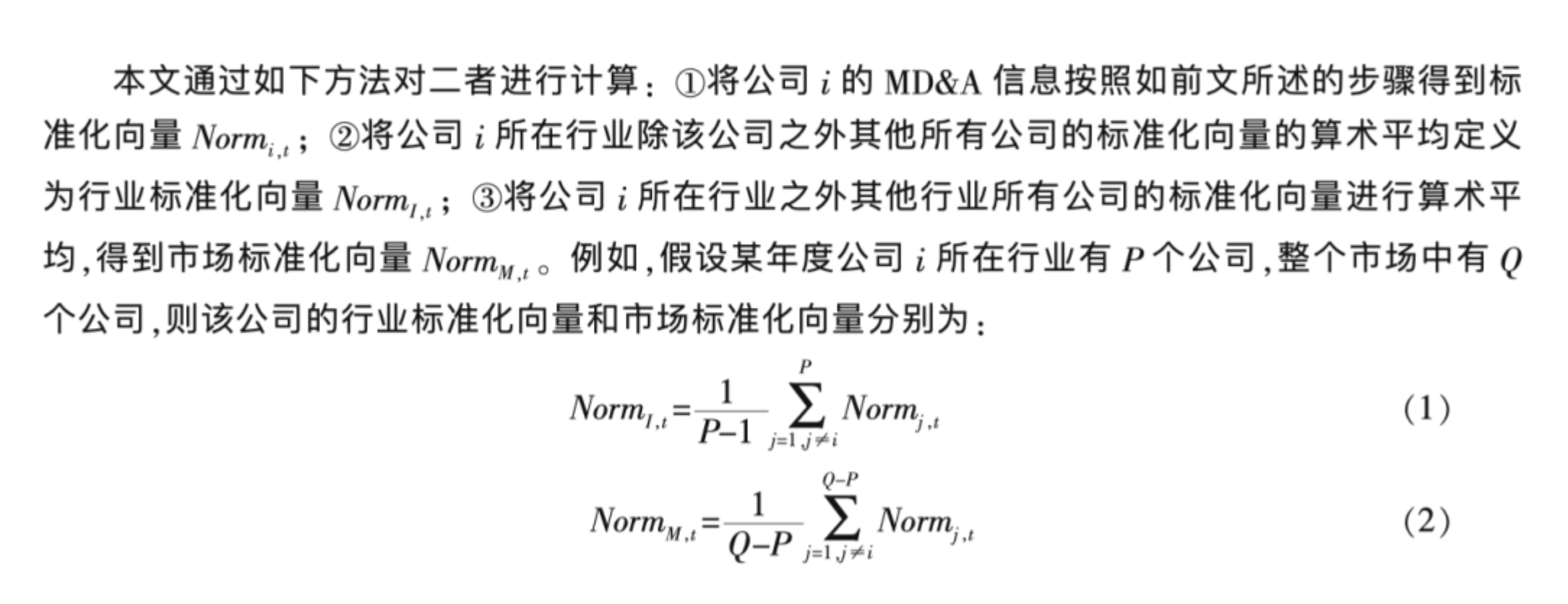from sklearn.feature_extraction.text import CountVectorizer
import numpy as np
import pandas as pd
import cntext as ct
import jieba
import os
import re

def transform(text):
#只保留md&a中的中文内容
text = ''.join(re.findall('[\u4e00-\u9fa5]+', text))
#剔除停用词
words = [w for w in jieba.cut(text) if w not in stopwords]
#整理为用空格间隔的字符串(类西方语言文本格式)
return ' '.join(words)

# converters 强制声明该列为字符串，  防止股票代码 被程序识别为数字，
#上市公司行业信息
df = pd.merge(df, ind_info_df, on=['股票代码', '会计年度'], how='inner')

# 剔除金融行业处理
df = df[~df['行业代码'].str.contains("J")]
df = df[~df['公司简称'].str.contains("ST")]

for year in [2010, 2011, 2012, 2013]:
#for year in set(df['会计年度'].values):
df_per_year = df[df['会计年度']==year]
df_per_year.reset_index(inplace=True)
df_per_year['clean_text'] = df_per_year['经营讨论与分析内容'].apply(transform)

cv = CountVectorizer(min_df=0.05, max_df=0.5)
# 生成稀疏bow矩阵
dtm_per_year = cv.fit_transform(df_per_year['clean_text'])
dtm_per_year = pd.DataFrame(dtm_per_year.toarray())
dtm_per_year = dtm_per_year.apply(lambda row: row/np.sum(row), axis=1)

dtm_per_year = pd.concat([df_per_year[['股票代码', '会计年度', '行业代码']], dtm_per_year], axis=1)

for idx in range(len(dtm_per_year)):
code = dtm_per_year.loc[idx, '股票代码']
ind = dtm_per_year.loc[idx, '行业代码']
year = dtm_per_year.loc[idx, '会计年度']

ind_freq = dtm_per_year[dtm_per_year['行业代码']==ind][dtm_per_year['股票代码']!=code].iloc[:, 3:].mean(axis=0)
market_freq = dtm_per_year[dtm_per_year['行业代码']!=ind].iloc[:, 3:].mean(axis=0)

dtm_per_year_melted = dtm_per_year.melt(id_vars=['股票代码', '会计年度', '行业代码'],
var_name='word_id',
value_name='word_freq')
corporate_df = pd.DataFrame({ 'word_id': dtm_per_year_melted[dtm_per_year_melted['股票代码']==code]['word_id'].values,
'word_freq': dtm_per_year_melted[dtm_per_year_melted['股票代码']==code]['word_freq'].values,
'ind_freq': ind_freq,
'market_freq':market_freq})
corporate_df['股票代码'] = code
corporate_df['行业代码'] = ind
corporate_df['会计年度'] = year
corporate_df.reset_index(inplace=True)
corporate_df = corporate_df[['股票代码', '行业代码', '会计年度', 'word_id', 'word_freq', 'ind_freq', 'market_freq']]
if not os.path.exists('output/{year}'.format(year=year)):
os.mkdir('output/{year}'.format(year=year))
corporate_df.to_csv('output/{year}/{code}.csv'.format(year=year, code=code), index=False)


## 五、标准信息、信息含量#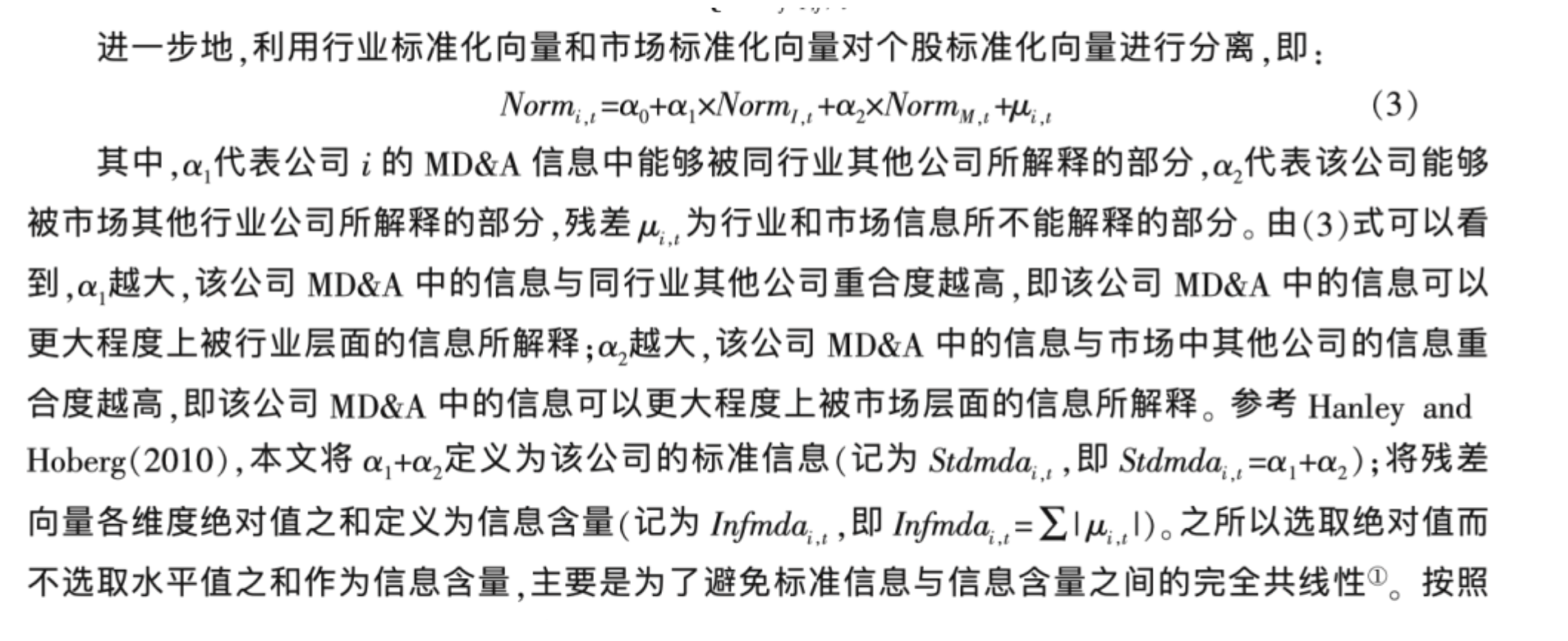import pandas as pd



Run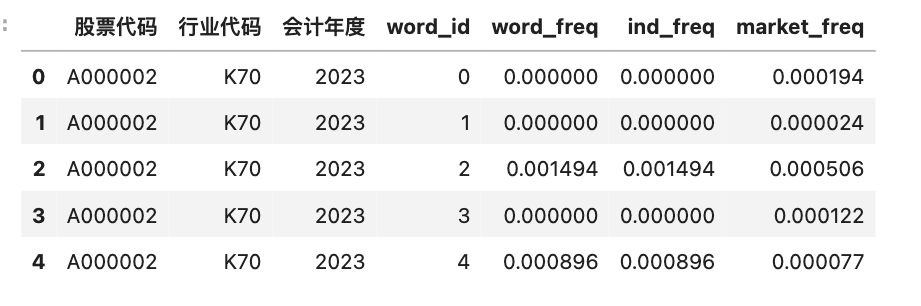#更改字段名
csv_df.columns = ['股票代码', '行业代码', '会计年度', 'word_id', 'Norm', 'Norm_Ind', 'Norm_Market']


Run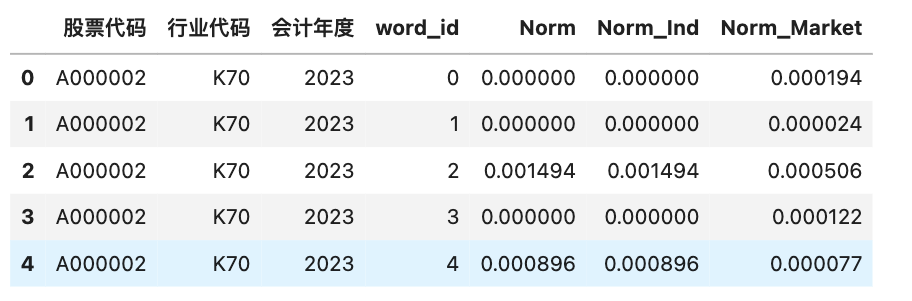import statsmodels.formula.api as smf

#因变量Norm
#解释变量Norm_Ind、 Norm_Market
formula = 'Norm ~ Norm_Ind + Norm_Market'

model = smf.ols(formula, data=csv_df)
result = model.fit()
print(result.summary())


Run


OLS Regression Results
==============================================================================
Dep. Variable:                   Norm   R-squared:                       0.319
Method:                 Least Squares   F-statistic:                     763.9
Date:                Fri, 06 Jan 2023   Prob (F-statistic):          6.88e-273
Time:                        06:37:27   Log-Likelihood:                 17334.
No. Observations:                3269   AIC:                        -3.466e+04
Df Residuals:                    3266   BIC:                        -3.464e+04
Df Model:                           2
Covariance Type:            nonrobust
===============================================================================
coef    std err          t      P>|t|      [0.025      0.975]
-------------------------------------------------------------------------------
Intercept    1.164e-05   2.86e-05      0.407      0.684   -4.44e-05    6.77e-05
Norm_Ind        0.7486      0.020     37.460      0.000       0.709       0.788
Norm_Market     0.2133      0.064      3.327      0.001       0.088       0.339
==============================================================================
Omnibus:                     4804.262   Durbin-Watson:                   2.026
Prob(Omnibus):                  0.000   Jarque-Bera (JB):          4165802.983
Skew:                           8.425   Prob(JB):                         0.00
Kurtosis:                     177.069   Cond. No.                     3.05e+03
==============================================================================

Notes:
 Standard Errors assume that the covariance matrix of the errors is correctly specified.
 The condition number is large, 3.05e+03. This might indicate that there are
strong multicollinearity or other numerical problems.


#标准信息
standard_info = result.params.Norm_Ind + result.params.Norm_Market

#信息含量
informative_content = sum(abs(result.resid))

print('000002标准信息: {}'.format(standard_info))
print('000002信息含量: {}'.format(informative_content))


Run

    000002标准信息: 0.9619640977801796
000002信息含量: 1.2750713760886476


import os
import csv
import statsmodels.formula.api as smf
import re

#结果存储到mda_infor.csv
with open('mda_infor.csv', 'a+', encoding='utf-8', newline='') as csvf:
fieldnames = ['股票代码', '会计年度', '标准信息', '信息含量']
writer = csv.DictWriter(csvf, fieldnames=fieldnames)

year_dirs = os.listdir('output')
year_dirs = [y for y in year_dirs if 'DS' not in y]
for year_dir in year_dirs:
code_csvfs = ['output/{year}/{csvf}'.format(year=year_dir, csvf=f)
for f in os.listdir('output/{}'.format(year_dir))]
code_csvfs = [f for f in code_csvfs if 'DS' not in f]
for csvf in code_csvfs:
try:
csv_df.columns = ['股票代码', '行业代码', '会计年度', 'word_id', 'Norm', 'Norm_Ind', 'Norm_Market']
formula = 'Norm ~ Norm_Ind + Norm_Market'
model = smf.ols(formula, data=csv_df)
result = model.fit()

#标准信息
standard_info = result.params.Norm_Ind + result.params.Norm_Market
#信息含量
informative_content = sum(abs(result.resid))

data = {'股票代码': re.findall('\d{6}', csvf),
'会计年度': re.findall('\d{4}', csvf),
'标准信息': standard_info,
'信息含量': informative_content}
writer.writerow(data)
except:
pass


## 最后#

import pandas as pd



Run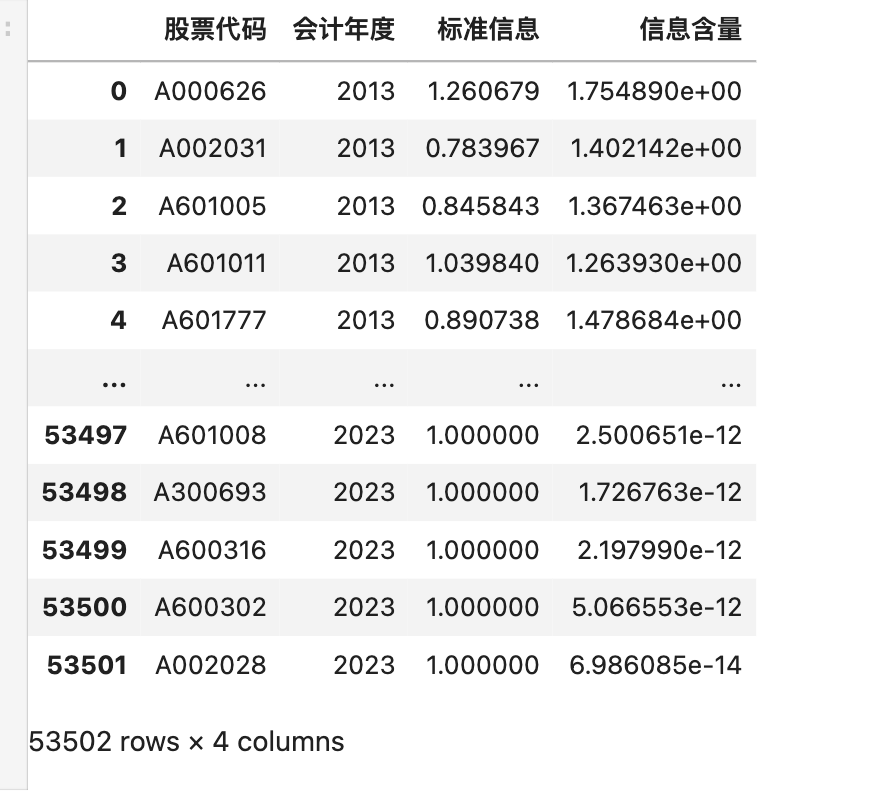mda_infor.csv含有2010-2020年的数据，如要复现原文，需要注意筛选数据。

print('mda_infor.csv记录数:',len(df))


Run

    mda_infor.csv记录数: 30173